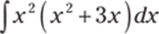﻿ ﻿The Fundamental Theorem of Calculus and the Net Change Theorem - The Questions - 1,001 Calculus Practice Problems

## The Questions

### The Fundamental Theorem of Calculus and the Net Change Theorem

Using Riemann sums to evaluate definite integrals (see Chapter 9) can be a cumbersome process. Fortunately, the fundamental theorem of calculus gives you a much easier way to evaluate definite integrals. In addition to evaluating definite integrals in this chapter, you start findingantiderivatives, or indefinite integrals. The net change theorem problems at the end of this chapter offer some insight into the use of definite integrals.

Although the antiderivative problems you encounter in this chapter aren't too complex, finding antiderivatives is in general a much more difficult process than finding derivatives, so consider yourself warned! You encounter many challenging antiderivative problems in later chapters.

The Problems You'll Work On

In this chapter, you see a variety of antiderivative problems:

· Finding derivatives of integrals

· Evaluating definite integrals

· Computing indefinite integrals

· Using the net change theorem to interpret definite integrals and to find the distance and displacement of a particle

What to Watch Out For

Although many of the problems in the chapter are easier antiderivative problems, you still need to be careful. Here are some tips:

· Simplify before computing the antiderivative. Don't forget to use trigonometric identities when simplifying the integrand.

· You don't often see problems that ask you to find derivatives of integrals, but make sure you practice them. They usually aren't that difficult, so they make for easier points on a quiz or test.

· Note the difference between distance and displacement; distance is always greater than or equal to zero, whereas displacement may be positive, negative, or zero! Finding the distance traveled typically involves more work than simply finding the displacement.

Using the Fundamental Theorem of Calculus to Find Derivatives

546–557 Find the derivative of the given function.

546.547.548.549.550.551.552.553.554.555.556.557.Working with Basic Examples of Definite Integrals

558–570 Evaluate the definite integral using basic antiderivative rules.

558.559.560.561.562.563.564.565.566.567.568.569., where570.Understanding Basic Indefinite Integrals

571–610 Find the indicated antiderivative.

571.572.573.574.575.576.577.578.579.580.581.582.583.584.585.586.587.588.589.590.591.592.593.594.595.596.597.598.599.600.601.602.603.604.605.606.607.608.609.610.Understanding the Net Change Theorem

611–619 Use the net change theorem to interpret the given definite integral.

611. If w'(t) is the rate of a baby's growth in pounds per week, what doesrepresent?

612. If r(t) represents the rate at which oil leaks from a tanker in gallons per minute, what doesrepresent?

613. A new bird population that is introduced into a refuge starts with 100 birds and increases at a rate of p'(t) birds per month. What doesrepresent?

614. If v(t) is the velocity of a particle in meters per second, what doesrepresent?

615. If a(t) is the acceleration of a car in meters per second squared, what doesrepresent?

616. If P'(t) represents the rate of production of solar panels, where t is measured in weeks, what doesrepresent?

617. The current in a wire I(t) is defined as the derivative of the charge, Q'(t) = I(t). What doesrepresent if t is measured in hours?

618. I'(t) represents the rate of change in your income in dollars from a new job, where t is measured in years. What doesrepresent?

619. Water is flowing into a pool at a rate of w'(t), where t is measured in minutes and w'(t) is measured in gallons per minute. What doesrepresent?

Finding the Displacement of a Particle Given the Velocity

620–624 A particle moves according to the given velocity function over the given interval. Find the displacement of the particle. Remember: Displacement is the change in position, and velocity is the rate of change in position with respect to time.

620. v(t) = 2t – 4, 0 ≤ t ≤ 5

621. v(t) = t2t – 6, 2 ≤ t ≤ 4

622. v(t) = 2 cos t, 0 ≤ t ≤ π

623. v(t) = sin t – cos t,624., 1 ≤ t ≤ 25

Finding the Distance Traveled by a Particle Given the Velocity

625–629 A particle moves according to the given velocity function over the given interval. Find the total distance traveled by the particle. Remember: Velocity is the rate of change in position with respect to time.

625. v(t) = 2t – 4, 0 ≤ t ≤ 5

626. v(t) = t2t – 6, 2 ≤ t ≤ 4

627. v(t) = 2 cos t, 0 ≤ t ≤ π

628. v(t) = sin t – cos t,629., 1 ≤ t ≤ 25

Finding the Displacement of a Particle Given Acceleration

630–632 A particle moves according to the given acceleration function over the given interval. First, find the velocity function. Then find the displacement of the particle. Remember: Displacement is the change in position, velocity is the rate of change in position with respect to time, and acceleration is the rate of change in velocity with respect to time.

630. a(t) = t + 2, v (0) = –6, 0 ≤ t ≤ 8

631. a(t) = 2t + 1, v (0) = –12, 0 ≤ t ≤ 5

632. a(t) = sin t + cos t,,Finding the Distance Traveled by a Particle Given Acceleration

633–635 A particle moves according to the given acceleration function over the given interval. Find the total distance traveled by the particle. Remember: Displacement is the change in position, velocity is the rate of change in position with respect to time, and acceleration is the rate of change in velocity with respect to time.

633. a(t) = t + 2, v (0) = –6, 0 ≤ t ≤ 8

634. a(t) = 2t + 1, v (0) = –12, 0 ≤ t ≤ 5

635. a(t) = sin t + cos t,,﻿